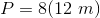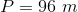# High School Math : How to find the perimeter of a polygon

## Example Questions

### Example Question #1 : How To Find The Perimeter Of A Polygon

What is the perimeter of a regular hendecagon with a side length of 32?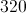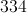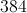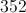Explanation:

To find the perimeter of a regular hendecagon you must first know the number of sides in a hendecagon is 11.

When you know the number of sides of a regular polygon to find the perimeter you must multiply the side length by the number of sides.

In this case it is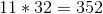.

The answer for the perimeter is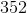### Example Question #1 : How To Find The Perimeter Of A Polygon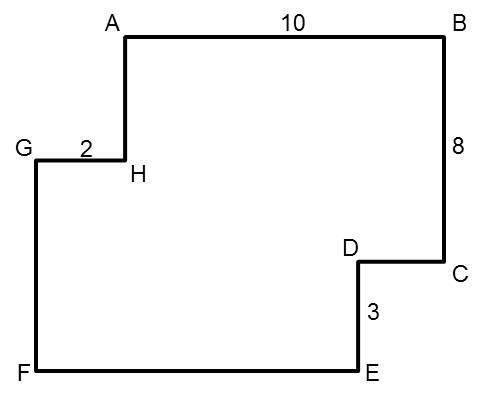All segments of the polygon meet at right angles (90 degrees). The length of segment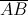is 10. The length of segment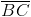is 8. The length of segment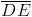is 3. The length of segment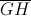is 2.

Find the perimeter of the polygon.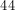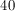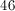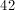Explanation:

The perimeter of the polygon is 46. Think of this polygon as a rectangle with two of its corners "flipped" inwards. This "flipping" changes the area of the rectangle, but not its perimeter; therefore, the top and bottom sides of the original rectangle would be 12 units long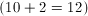. The left and right sides would be 11 units long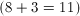. Adding all four sides, we find that the perimeter of the recangle (and therefore, of this polygon) is 46.

### Example Question #2 : How To Find The Perimeter Of A Polygon

What is the perimeter of a regular nonagon with a side length of?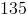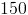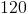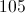Explanation:

To find the perimeter of a regular polygon, we take the length of each side,, and multiply it by the number of sides,.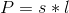In a nonagon the number of sides is, and in this example the side length is.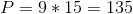The perimeter is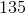.

### Example Question #3 : How To Find The Perimeter Of A Polygon

Find the perimeter of the following octagon: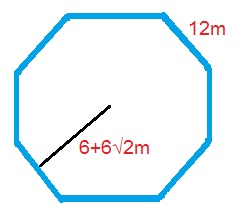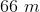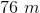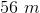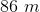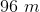Explanation:

The formula for the perimeter of an octagon is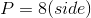.

Plugging in our values, we get: